## Tuesday, April 28, 2015

### plotTree.wBars with tip labels

I have just submitted a new version of phytools (0.4-56) to CRAN. Fingers crossed that it survives scrutiny & is accepted; however in the meantime it can be downloaded from the phytools page.

In a different post I will detail the updates (which are many) from both the previous CRAN version and from other recent non-CRAN phytools releases; however one feature that has been added is a modification to the phytools function `plotTree.wBars` to permit tip labels to be plotted. This function adds bars for a continuous trait to the tips of the tree. Here is a quick demo with the default conditions:

``````library(phytools)
packageVersion("phytools")
``````
``````##  '0.4.56'
``````
``````## make realistic looking tip labels
tip.label<-replicate(60,paste(sample(LETTERS,1),"._",
paste(sample(letters,round(runif(n=1,min=4,max=8))),
collapse=""),sep=""))
## simulate tree
tree<-pbtree(n=60,tip.label=tip.label,scale=1)
## simulate positive trait data - negative values are
## permitted, and I will show that below
x<-abs(fastBM(tree))
## finally, plot without tree labels
plotTree.wBars(tree,x,scale=0.2)
``````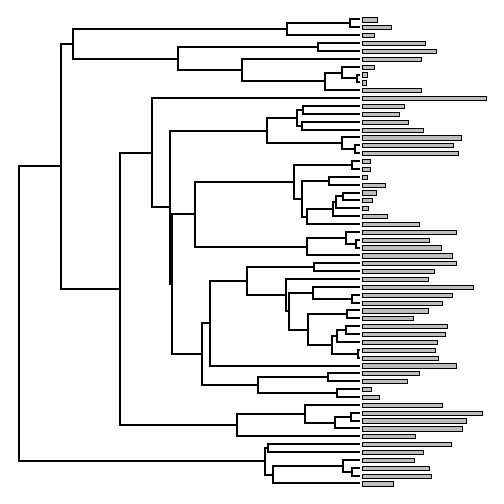Although I received lots of positive feedback about this function, users wanted to be able to include tip labels (very reasonably, I must admit). The only added complication with this is that we now have to compute not only how much space we need for the phylogeny & the trait data to be plotted, but also how much space is required to plot the tip labels. This is more complicated that it sounds, even, because the amount of space required for the tip labels cannot be found without first creating the plotting device.

The code for the updated version of `plotTree.wBars` is here.

Here is how it looks, for the same data & tree:

``````plotTree.wBars(tree,x,scale=0.2,tip.labels=TRUE,fsize=0.6)
``````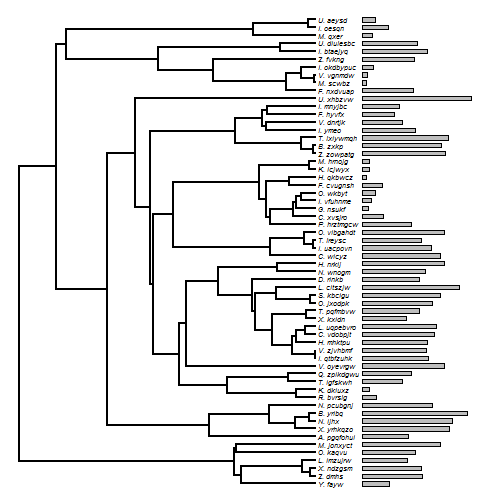For larger trees especially, we may want to plot our tree in a fan style. This also works with tip labels, for instance as follows:

``````tip.label<-replicate(200,paste(sample(LETTERS,1),"._",
paste(sample(letters,round(runif(n=1,min=4,max=8))),
collapse=""),sep=""))
tree<-pbtree(n=200,tip.label=tip.label,scale=1)
x<-abs(fastBM(tree))
plotTree.wBars(tree,x,fsize=0.4,scale=0.1,tip.labels=TRUE,type="fan",lwd=1)
``````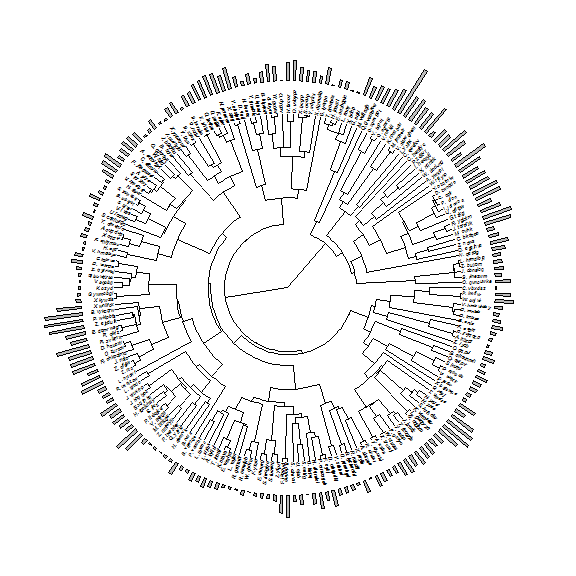I'm sure I've demonstrated this before, but it is fairly straightforward to combine `plotTree.wBars` with other phytools plotting methods, such as `contMap` - continuous character mapping onto the tree. So for example:

``````## create an object of class "contMap"
obj<-contMap(tree,x,plot=FALSE)
plotTree.wBars(obj\$tree,x,fsize=0.4,scale=0.1,tip.labels=TRUE,
type="fan",method="plotSimmap",colors=obj\$cols)
prompt=FALSE,x=0.9*par()\$usr,y=0.9*par()\$usr)
``````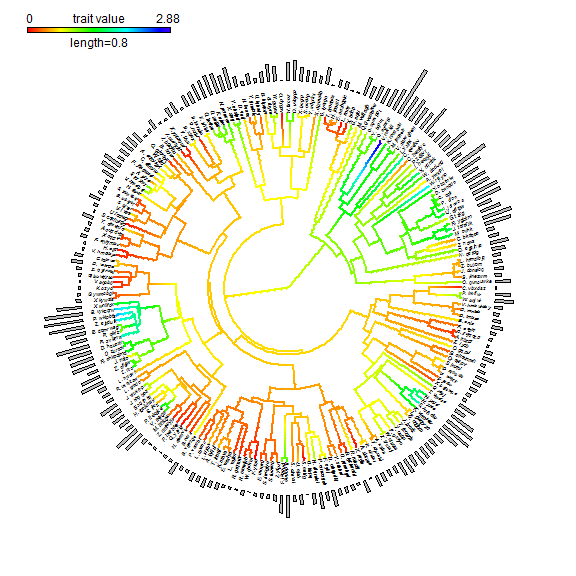You might notice lots of 'aliasing' in this plot. Obviously, we wouldn't want this for publication. We can easily avoid it by exporting to PDF or other lossless formats from within R. In this case, we could simply do:

``````pdf(file="28Apr15-post.pdf")
plotTree.wBars(obj\$tree,x,fsize=0.4,scale=0.1,tip.labels=TRUE,
type="fan",method="plotSimmap",colors=obj\$cols)
prompt=FALSE,x=0.9*par()\$usr,y=0.9*par()\$usr)
dev.off()
``````
``````## png
##   2
``````

You can see this product here: 28Apr15-post.pdf.

Finally, we can plot negative values. So, again with a smaller tree:

``````tip.label<-replicate(60,paste(sample(LETTERS,1),"._",
paste(sample(letters,round(runif(n=1,min=4,max=8))),
collapse=""),sep=""))
tree<-pbtree(n=60,tip.label=tip.label,scale=1)
x<-fastBM(tree)
plotTree.wBars(tree,x,scale=0.2,tip.labels=TRUE,fsize=0.6)
``````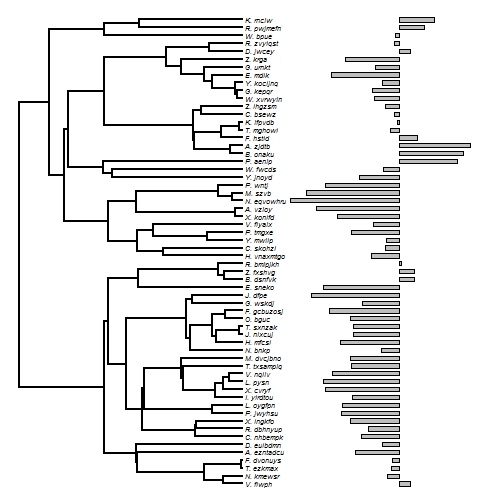``````## or
obj<-contMap(tree,x,plot=FALSE)
plotTree.wBars(obj\$tree,x,fsize=0.6,scale=0.2,tip.labels=TRUE,
method="plotSimmap",colors=obj\$cols)
``````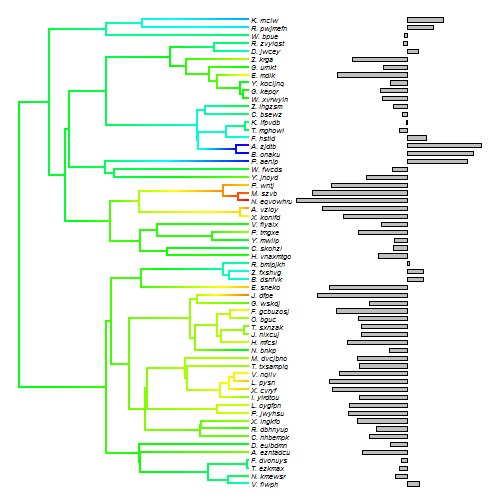OK, that's it.

1.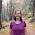Great function! Anyway to color the tip labels? I tried tip.color = color_vector, but did not work. Thanks!

2.There is a manner to show the family name (for each family group) instead the tip label? Cheers

1.You could try using the function cladelabels (e.g., here).

3.Cool function!

There is any way of connecting the tiplabels with the bars?
Like a dotted line or similar?

I am working with a huge tree and have some visualization problems.

If I want to see the bars without zoom it I have to plot them so wide
(what it´s totally fie for a printed version).

The problem is that for a digital version when I zoom the tree,
it´s difficult to properly identify the genus that connect to each bar, because are too wide. And I can only use a single plot for both printed and digital.

One solution can be printing some target tip labels in bold, so when I zoom I can identify the target genus.
Another solution would be connecting target tip labels with their bars with a dotted line or so...

Cheers,

Julia

4.Hi Liam,

I have made a phylo tree with the plotTree.wBars. But the tree was very dense in some clade. I want to get a tree with cladogram-transform branch. How could I get this tree?

Note: due to the very large amount of spam, all comments are now automatically submitted for moderation.